Скачать презентацию MAE 5360 Hypersonic Airbreathing Engines Rayleigh Flow and

7b5a5b89c92ac9662874981171c5bb1d.ppt

• Количество слайдов: 29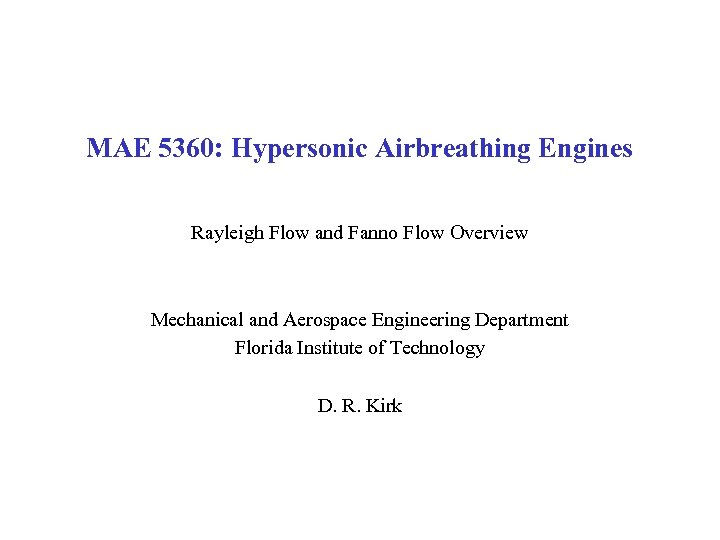MAE 5360: Hypersonic Airbreathing Engines Rayleigh Flow and Fanno Flow Overview Mechanical and Aerospace Engineering Department Florida Institute of Technology D. R. Kirk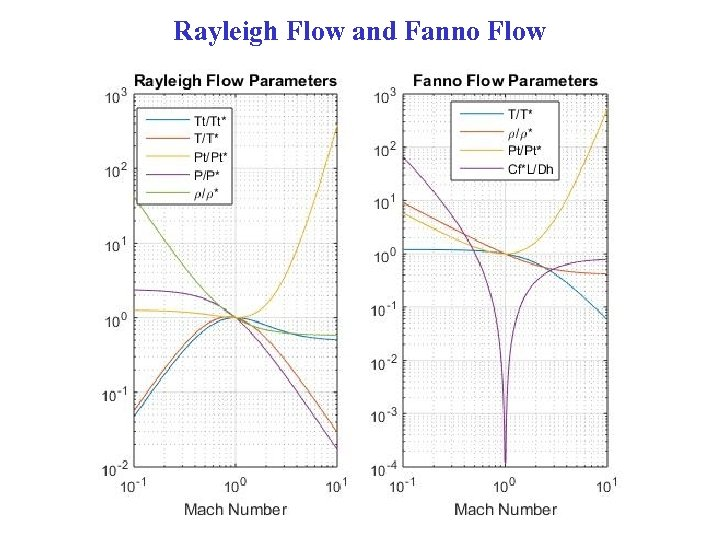Rayleigh Flow and Fanno Flow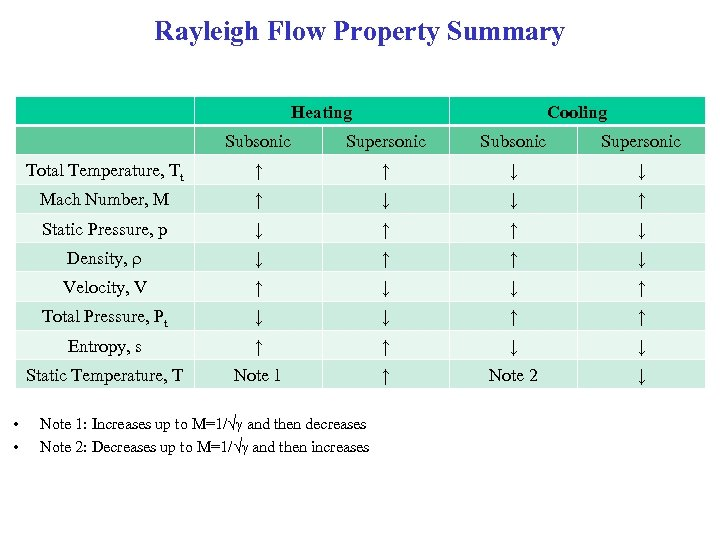Rayleigh Flow Property Summary Heating Cooling Subsonic Supersonic Total Temperature, Tt ↑ ↑ ↓ ↓ Mach Number, M ↑ ↓ ↓ ↑ Static Pressure, p ↓ ↑ ↑ ↓ Density, r ↓ ↑ ↑ ↓ Velocity, V ↑ ↓ ↓ ↑ Total Pressure, Pt ↓ ↓ ↑ ↑ Entropy, s ↑ ↑ ↓ ↓ Static Temperature, T • • Supersonic Note 1 ↑ Note 2 ↓ Note 1: Increases up to M=1/√g and then decreases Note 2: Decreases up to M=1/√g and then increases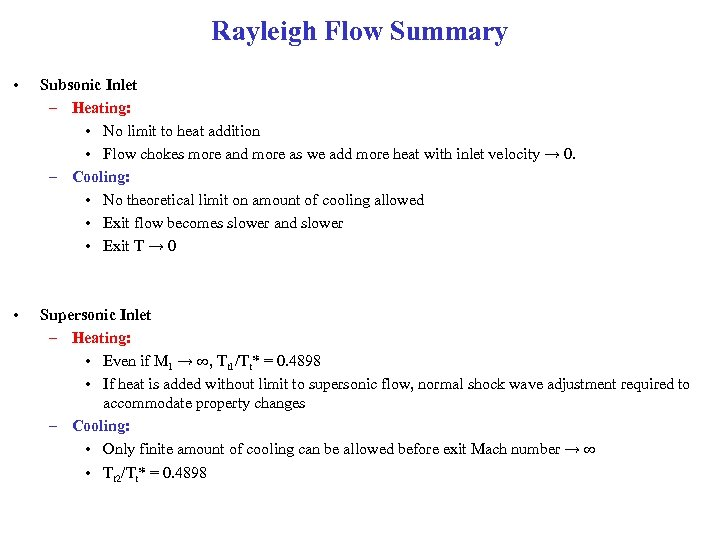Rayleigh Flow Summary • Subsonic Inlet – Heating: • No limit to heat addition • Flow chokes more and more as we add more heat with inlet velocity → 0. – Cooling: • No theoretical limit on amount of cooling allowed • Exit flow becomes slower and slower • Exit T → 0 • Supersonic Inlet – Heating: • Even if M 1 → ∞, Tt 1/Tt* = 0. 4898 • If heat is added without limit to supersonic flow, normal shock wave adjustment required to accommodate property changes – Cooling: • Only finite amount of cooling can be allowed before exit Mach number → ∞ • Tt 2/Tt* = 0. 4898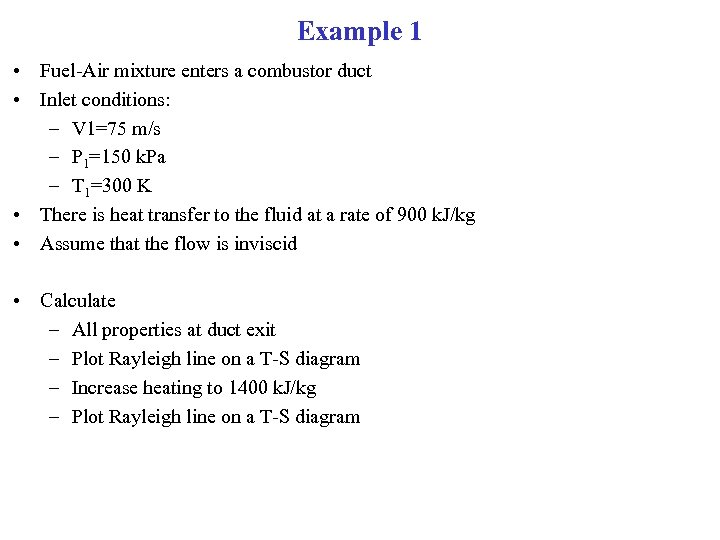Example 1 • Fuel-Air mixture enters a combustor duct • Inlet conditions: – V 1=75 m/s – P 1=150 k. Pa – T 1=300 K • There is heat transfer to the fluid at a rate of 900 k. J/kg • Assume that the flow is inviscid • Calculate – All properties at duct exit – Plot Rayleigh line on a T-S diagram – Increase heating to 1400 k. J/kg – Plot Rayleigh line on a T-S diagram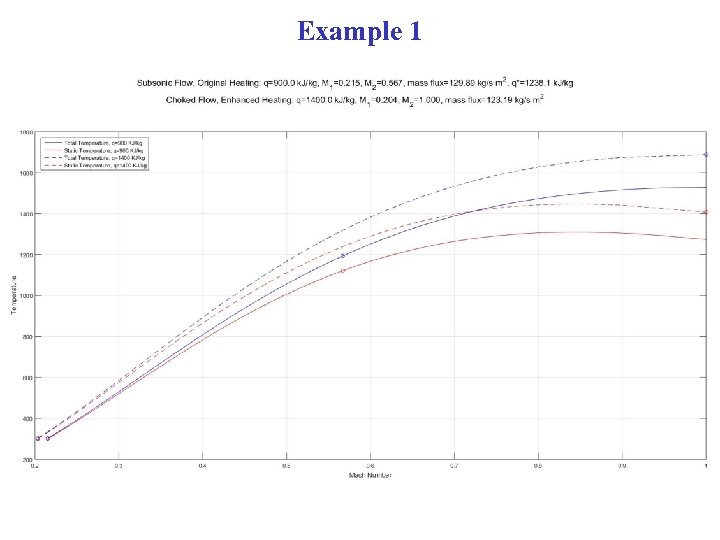Example 1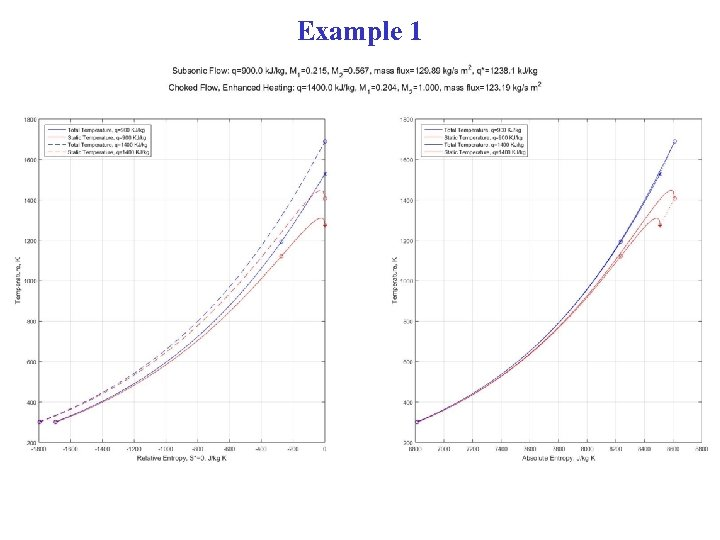Example 1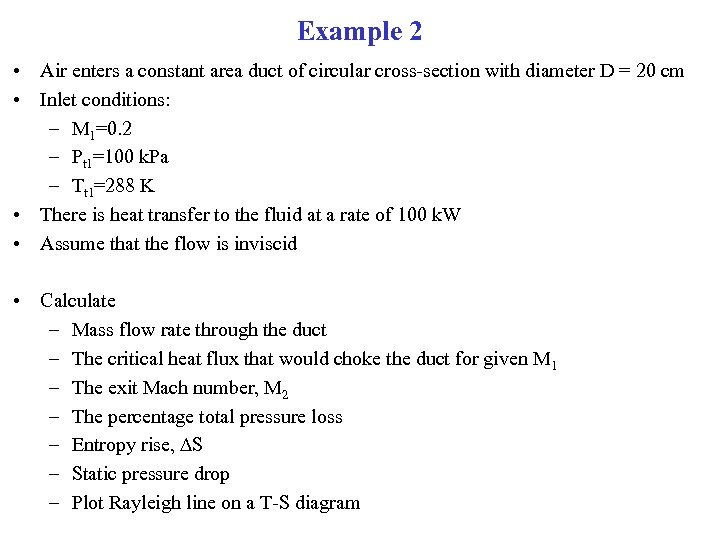Example 2 • Air enters a constant area duct of circular cross-section with diameter D = 20 cm • Inlet conditions: – M 1=0. 2 – Pt 1=100 k. Pa – Tt 1=288 K • There is heat transfer to the fluid at a rate of 100 k. W • Assume that the flow is inviscid • Calculate – Mass flow rate through the duct – The critical heat flux that would choke the duct for given M 1 – The exit Mach number, M 2 – The percentage total pressure loss – Entropy rise, DS – Static pressure drop – Plot Rayleigh line on a T-S diagram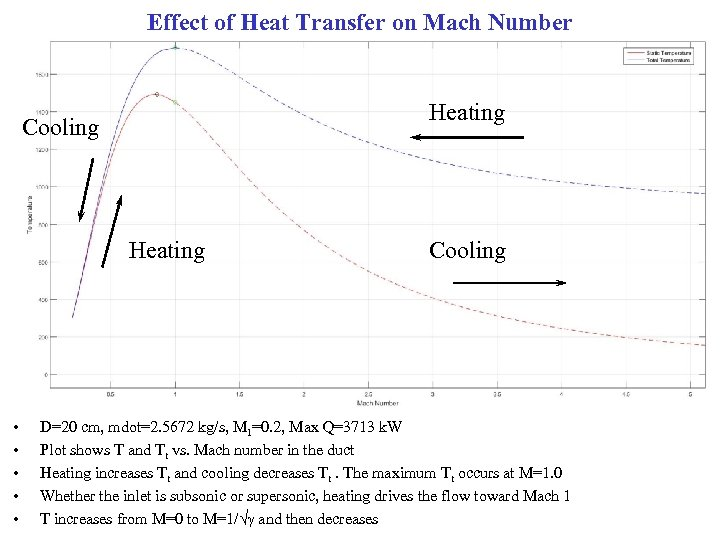Effect of Heat Transfer on Mach Number Heating Cooling Heating • • • Cooling D=20 cm, mdot=2. 5672 kg/s, M 1=0. 2, Max Q=3713 k. W Plot shows T and Tt vs. Mach number in the duct Heating increases Tt and cooling decreases Tt. The maximum Tt occurs at M=1. 0 Whether the inlet is subsonic or supersonic, heating drives the flow toward Mach 1 T increases from M=0 to M=1/√g and then decreases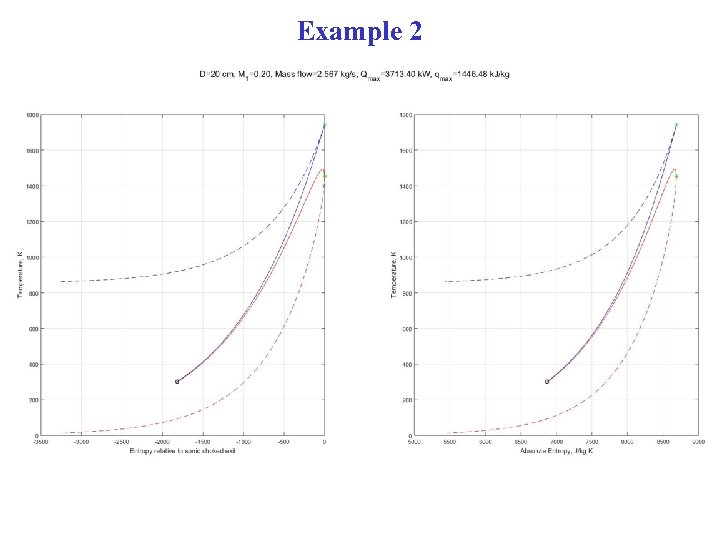Example 2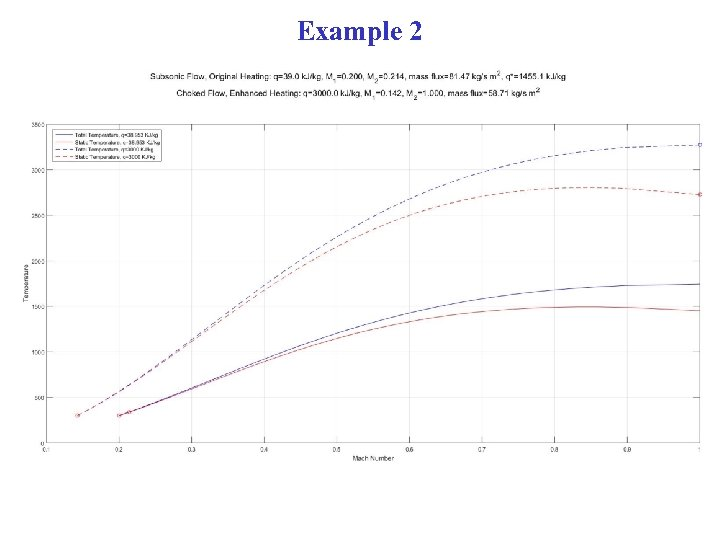Example 2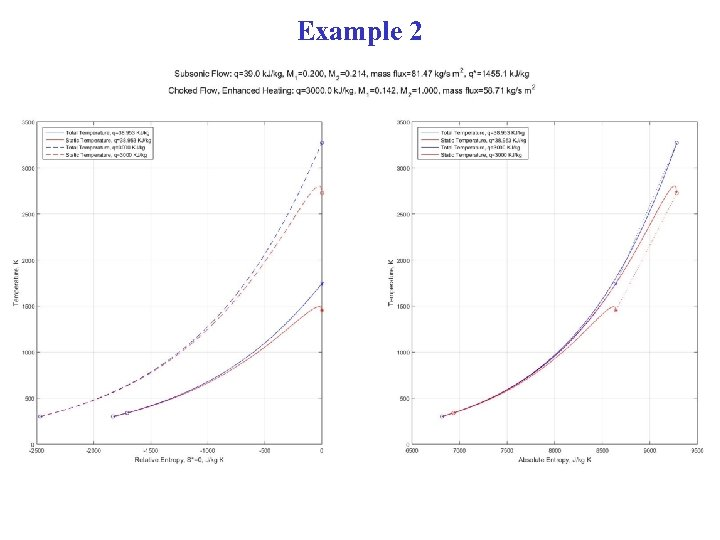Example 2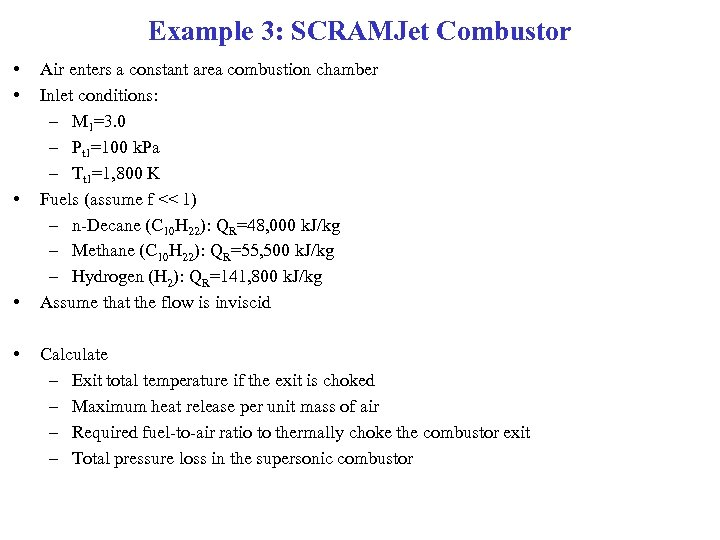Example 3: SCRAMJet Combustor • • • Air enters a constant area combustion chamber Inlet conditions: – M 1=3. 0 – Pt 1=100 k. Pa – Tt 1=1, 800 K Fuels (assume f << 1) – n-Decane (C 10 H 22): QR=48, 000 k. J/kg – Methane (C 10 H 22): QR=55, 500 k. J/kg – Hydrogen (H 2): QR=141, 800 k. J/kg Assume that the flow is inviscid Calculate – Exit total temperature if the exit is choked – Maximum heat release per unit mass of air – Required fuel-to-air ratio to thermally choke the combustor exit – Total pressure loss in the supersonic combustor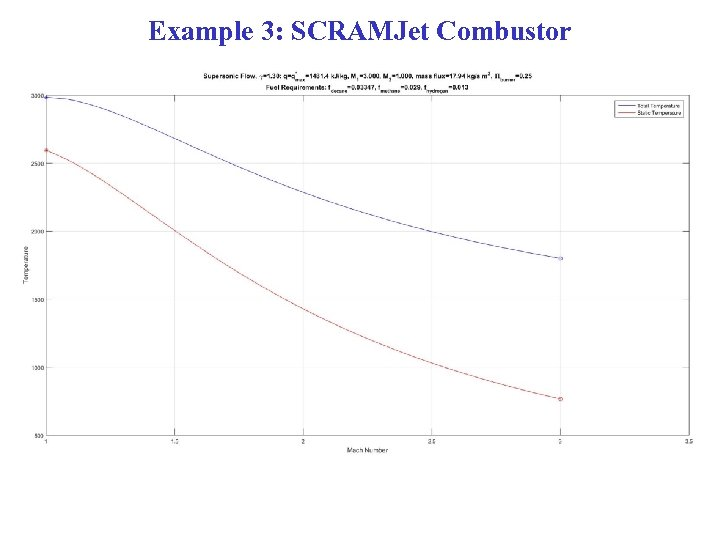Example 3: SCRAMJet Combustor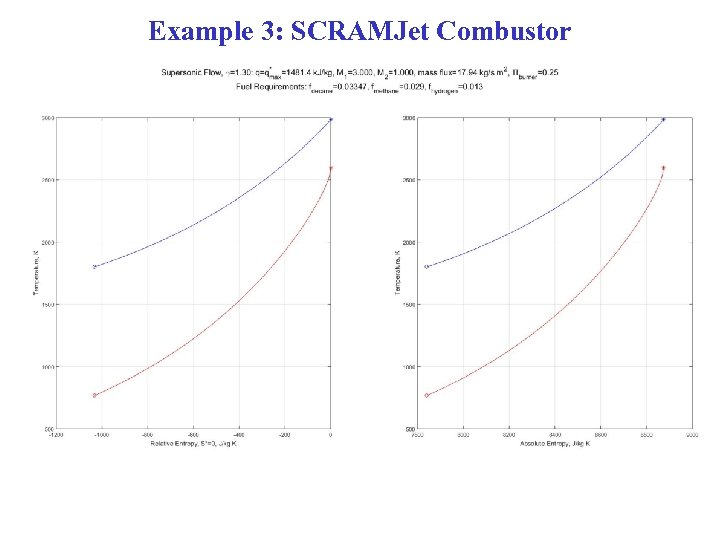Example 3: SCRAMJet CombustorRayleigh Flow and Fanno Flow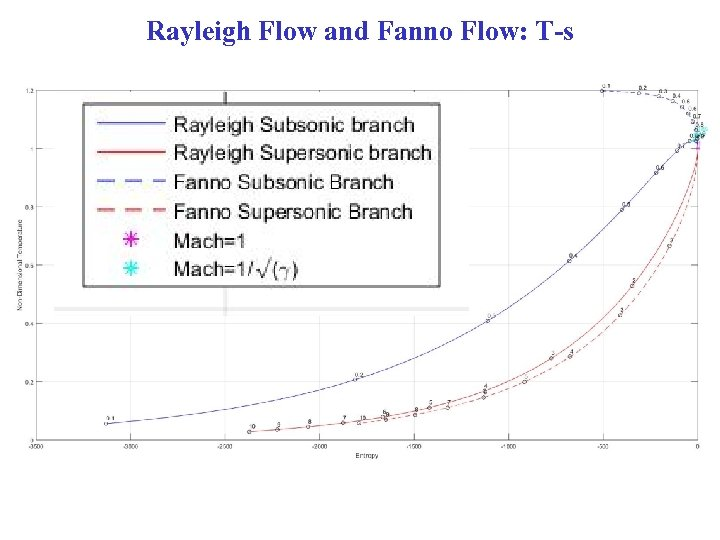Rayleigh Flow and Fanno Flow: T-s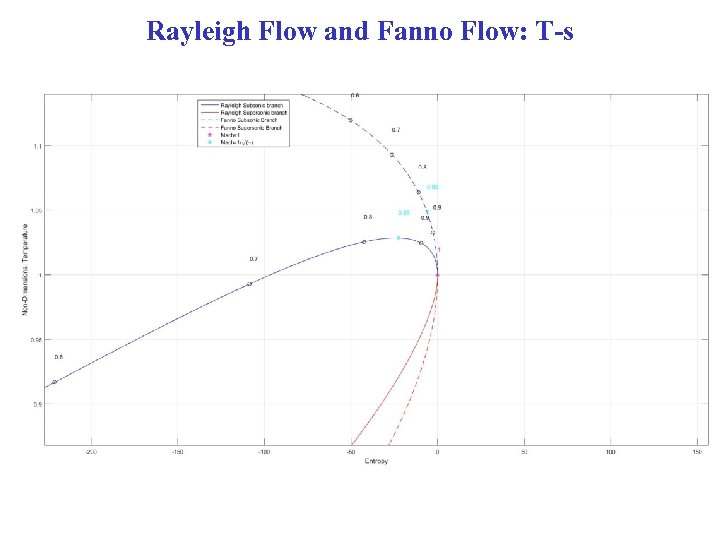Rayleigh Flow and Fanno Flow: T-s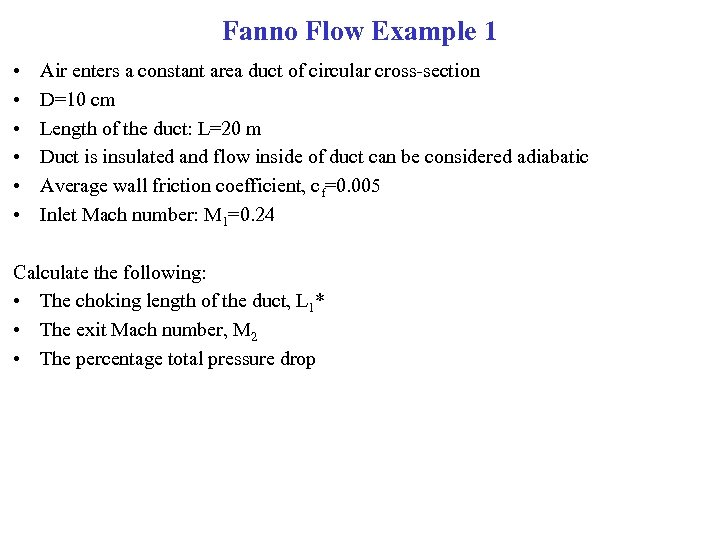Fanno Flow Example 1 • • • Air enters a constant area duct of circular cross-section D=10 cm Length of the duct: L=20 m Duct is insulated and flow inside of duct can be considered adiabatic Average wall friction coefficient, cf=0. 005 Inlet Mach number: M 1=0. 24 Calculate the following: • The choking length of the duct, L 1* • The exit Mach number, M 2 • The percentage total pressure drop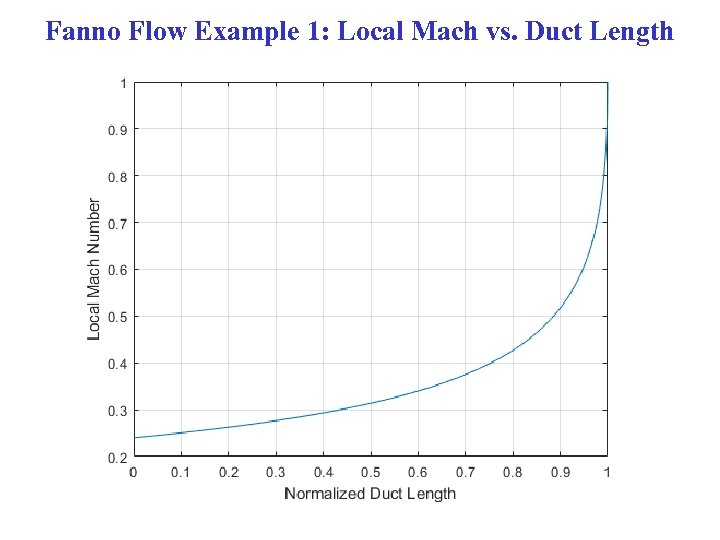Fanno Flow Example 1: Local Mach vs. Duct Length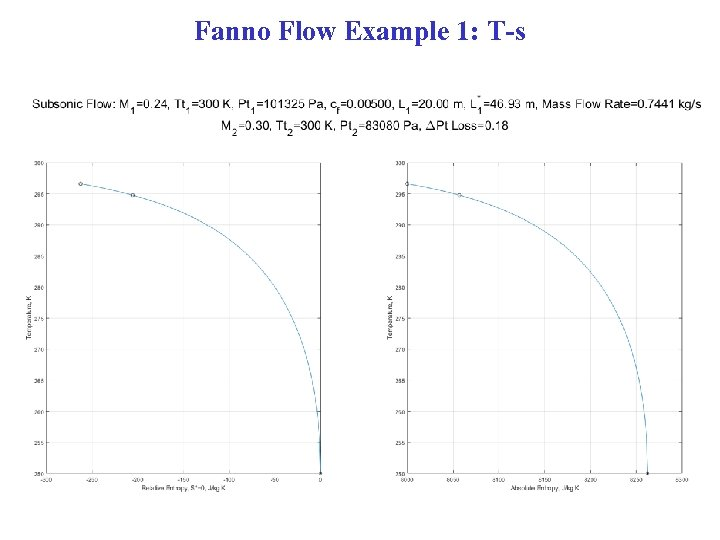Fanno Flow Example 1: T-s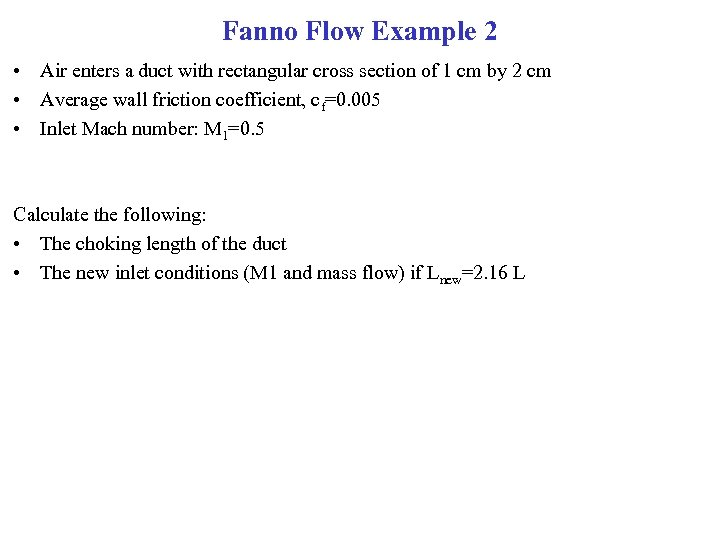Fanno Flow Example 2 • Air enters a duct with rectangular cross section of 1 cm by 2 cm • Average wall friction coefficient, cf=0. 005 • Inlet Mach number: M 1=0. 5 Calculate the following: • The choking length of the duct • The new inlet conditions (M 1 and mass flow) if Lnew=2. 16 L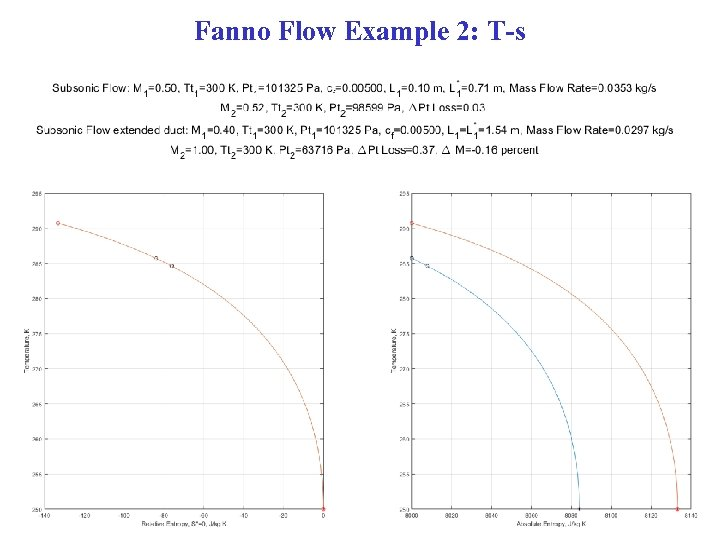Fanno Flow Example 2: T-s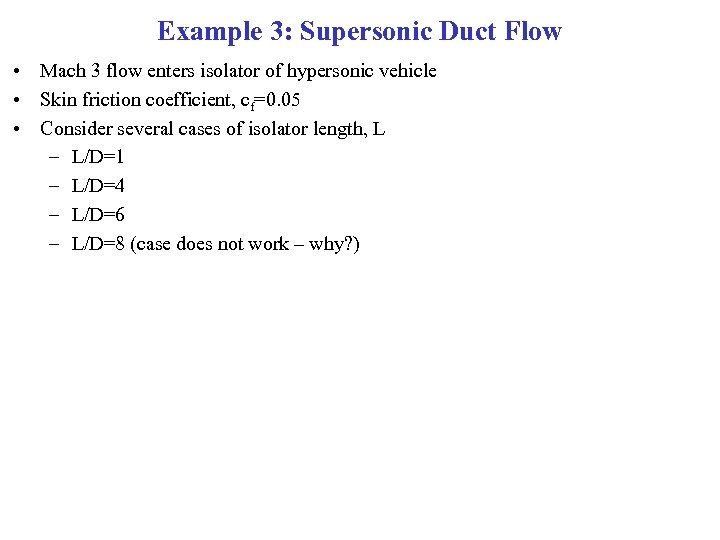Example 3: Supersonic Duct Flow • Mach 3 flow enters isolator of hypersonic vehicle • Skin friction coefficient, cf=0. 05 • Consider several cases of isolator length, L – L/D=1 – L/D=4 – L/D=6 – L/D=8 (case does not work – why? )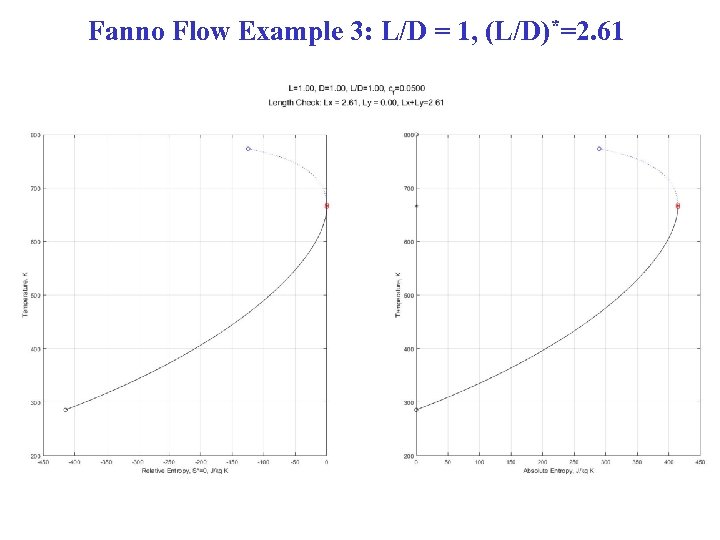Fanno Flow Example 3: L/D = 1, (L/D)*=2. 61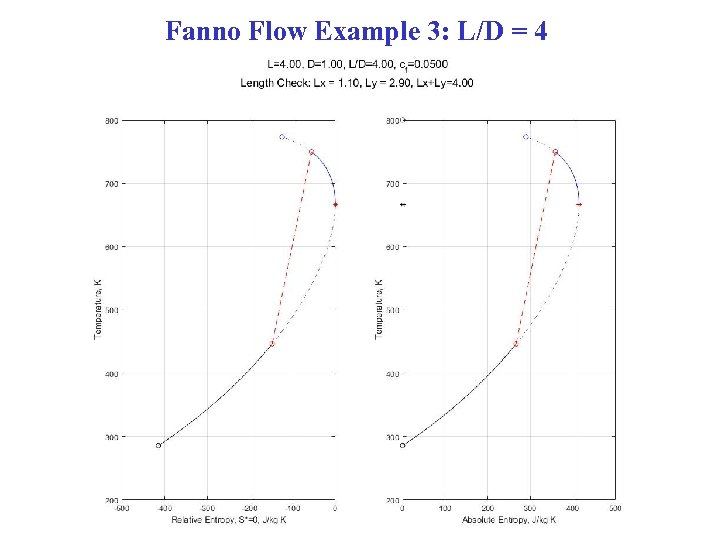Fanno Flow Example 3: L/D = 4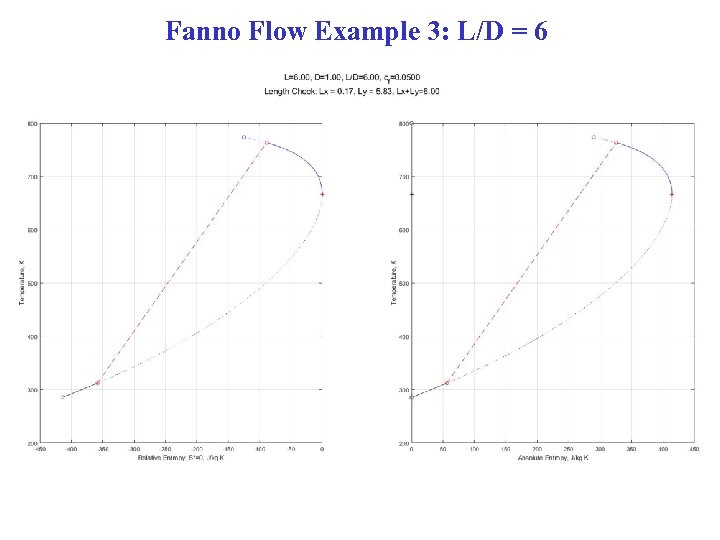Fanno Flow Example 3: L/D = 6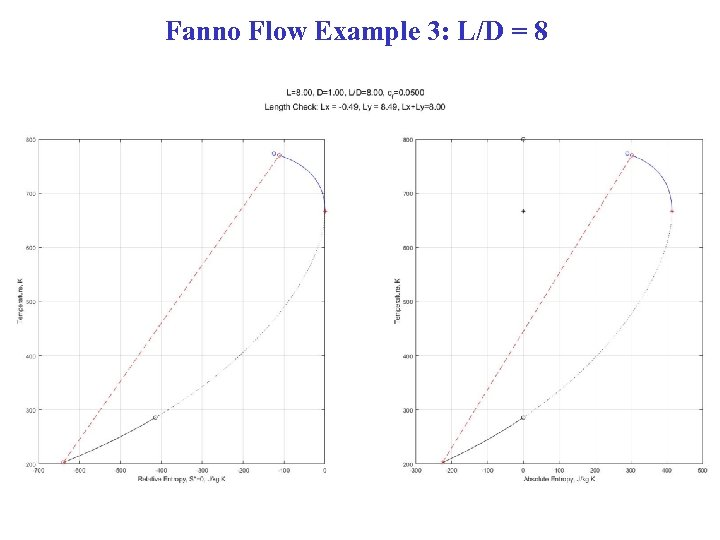Fanno Flow Example 3: L/D = 8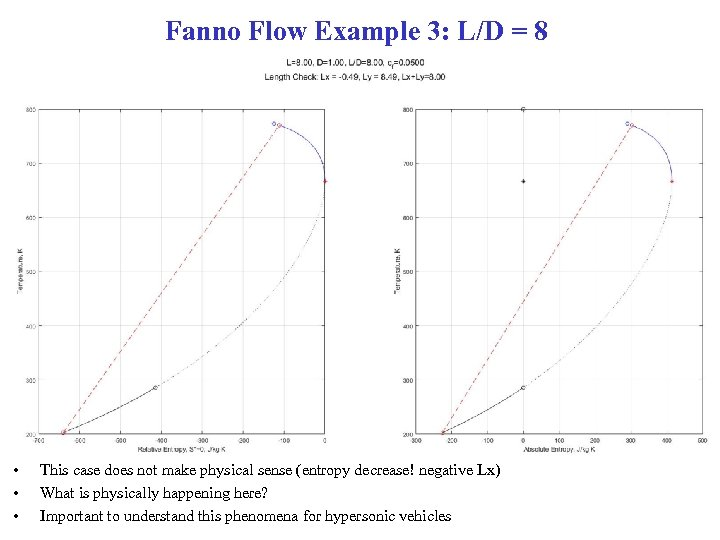Fanno Flow Example 3: L/D = 8 • • • This case does not make physical sense (entropy decrease! negative Lx) What is physically happening here? Important to understand this phenomena for hypersonic vehicles